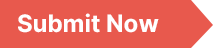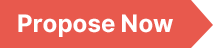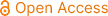### Applied and Computational Mathematics

Archive
• 2023, Volume 12

• 2022, Volume 11

• 2021, Volume 10

• 2020, Volume 9

• 2019, Volume 8

• 2018, Volume 7

• 2017, Volume 6

• 2016, Volume 5

• 2015, Volume 4

• 2014, Volume 3

• 2013, Volume 2

• 2012, Volume 1

Submit a Manuscript

Publishing with us to make your research visible to the widest possible audience.Propose a Special Issue

Building a community of authors and readers to discuss the latest research and develop new ideas.Research Article |### Trapping Issues for Weight-dependent Walks in the Weighted Extended Cayley Networks

The weighted extended Cayley networks are an extension of extended Cayley networks, which are the structures constructed by introducing power spaces into traditional Cayley trees. The weighted extended Cayley networks are constructed depending on two structural parameters of the network m, n and a weight factor r. Firstly, we used a new calculation method to calculate the exact analytic formula of the average weighted shortest path (AWSP). The obtained results show that: (1) For very large systems, the AWSPs for different value of weight factor r are less affected by the parameter m. (2) The AWSPs are less affected by the weight factor r when r is greater than 0 and less than or equal to n, while the AWSPs depend on the scaling factor r when r is greater than n. We have presented a trapping issue of weight-dependent walks in the weighted extended Cayley networks, focusing on a specific case with a perfect trap located at the central node. Then, the scaling expression of the average trapping time (ATT) is derived based on the layering of weighted extended Cayley networks. It was surprisingly found that (1) Regardless of the relationship between m and n, the dominant terms of ATTs are consistent. (2) ATTs are less affected by the structural parameter m and the weight factor r when r is less than or equal to the ratio of n to m−1, indicating that the efficiency of the trapping process is independent of m and r. (3) When r is greater than the ratio of n to m−1, the efficiency of the trapping process depends on three main parameters: two structural parameters of the networks m, n and a weight factor r, which means that the smaller the multiplier of three numbers r, n and m − 1 is, the more efficient the trapping process is. Therefore, the trapping efficiency of the weighted extended Cayley networks is not only affected by the underlying structures of the networks m and n, but also by the weight factor r.

Trapping Time, Average Weighted Shortest Path, Weighted Extended Cayley Networks, Weight-dependent Walk

Dandan Ye, Fei Zhang, Yiteng Qin, Xiaojuan Zhang, Ning Zhang, et al. (2023). Trapping Issues for Weight-dependent Walks in the Weighted Extended Cayley Networks. Applied and Computational Mathematics, 12(5), 114-139. https://doi.org/10.11648/j.acm.20231205.12